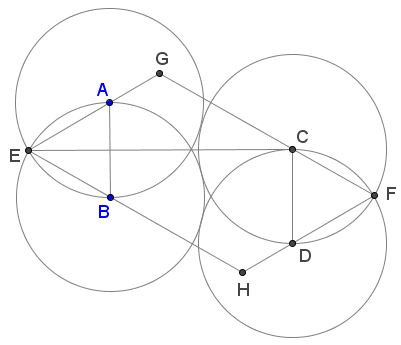# Four Golden Circles

Bùi Quang Tuån has posted on the CutTheKnotMath facebook page a configuration of four circles and a parallelogram rich in interrelations between the parts among which are several sightings of the Golden Ratio.

### Main statement

Each of the four congruent circles $(A),$ $(B),$ $(C),$ $(D)$ touches other two and passes through the center of the remaining third, as shown:In the parallelogram $EHFG,$

1. The sides are in Golden Ratio: $FG/EG=\phi,$

2. The diagonals are in Golden Ratio: $EF/GH=\phi,$

3. Circle centers divide the sides in Golden Ratio, e.g., $AE/AG=BE/BH=\phi,$

4. The two center lines $AB$ and $CD$ are divided in Golden Ratio by the diagonal $EF,$ e.g. $AI/BI=\phi,$

5. Two lines $BF$ and $CE$ are divided in Golden Ratio by the diagonal $GH,$ e.g. $EK/CK=\phi,$

6. In turn, lines $BF$ and $CE$ divide the diagonal $GH$ in Golden Ratio, e.g., $GL/HL=\phi.$

Note: The configuration has several additional properties worth of consideration. These are listed at the bottom of the page.

### Proof

Without loss of generality, assume that the common radius of the four circles is $1.$ Let $N$ be the second intersection of $(A)$ and $(B);$ $M$ the midpoint of $AB.$Due to the symmetry of the configuration, $M$ is the intersection of $AB$ and $CE,$ $\Delta ABC$ is isosceles, with $AB=1,$ $AC=BC=2.$ By the Pythagorean theorem, $CM=\sqrt{15}/2.$ In equilateral $\Delta ABE,$ sides are equal to $1$ so that $EM = \sqrt{3}/2.$ By symmetry, also $MN=\sqrt{3}/2.$ Thus

$\displaystyle CE=\frac{\sqrt{15}}{2}+\frac{\sqrt{3}}{2}=\frac{\sqrt{3}}{2}(\sqrt{5}+1)$

and $\displaystyle\frac{CE}{EN}=\frac{\sqrt{5}+1}{2}=\phi.$

Let now $I$ and $J$ be the intersections of $EF$ with $AB$ and $CD,$ respectively. By symmetry, $AI=DJ,$ $BI=CJ.$Triangles $CEJ$ and $MEI$ are similar so that $CE/EM=CJ/MI.$ From the foregoing discussion, $CE/EM=\sqrt{5}+1.$ Let $CJ=BI=x.$ Then $\displaystyle MI=\frac{1}{2}-x,$ giving an equation:

$\displaystyle\frac{x}{\frac{1}{2}-x}=\sqrt{5}+1,$

solving which we get $\displaystyle BI=x=\frac{3-\sqrt{5}}{2}.$ Now, $\displaystyle AI=1-x=\frac{\sqrt{5}-1}{2}$ and, as can be easily verified, $\displaystyle\frac{AI}{BI}=\phi.$ This proves #4.

Further, $\angle BEA=60^{\circ}$ so $\angle EGC=\angle EGF=120^{\circ}.$$\Delta EGC$ is isosceles, with $\angle CEG=30^{\circ},$ implying

\begin{align}\displaystyle EG &=\frac{\frac{1}{2}CE}{\cos 30^{\circ}}\\ &=\frac{1}{2}\cdot \frac{\sqrt{3}}{2}(\sqrt{5}+1)\cdot\frac{2}{\sqrt{3}}\\ &=\frac{\sqrt{5}+1}{2}=\phi. \end{align}

$FG=GC+CF=\phi +1=\phi^{2}$ such that $FG/EG=FG/CG=\phi,$ proving #1. We now possess all segment lengths to verify #3 directly.

The validity of #4 is the subject of the following

### Lemma

In a parallelogram $EHFG$ with angles of $60^{\circ}$ and $120^{\circ},$ if the sides relate in the Golden Ratio, so do the diagonals.

### Proof

Using coordinates makes a 1-step proof.Let $E$ be the origin, $G=(1,0),$ $EH=\phi,$ and $\angle GEH=60^{\circ}.$ Then $\displaystyle H=\bigg(\frac{\phi}{2},\frac{\phi\sqrt{3}}{2}\bigg),$ implying

\begin{align}\displaystyle GH^{2}&=\bigg(1-\frac{\phi}{2}\bigg)^{2}+\bigg(\frac{\phi\sqrt{3}}{2}\bigg)^{2}\\ &=1-\phi+\frac{\phi^{2}}{4}+\frac{3\phi^{2}}{4}\\ &=1-\phi+\phi^{2}\\ &=(1-\phi)+(1+\phi)=2. \end{align}

Similarly, $EF^{2}=(1+\phi)+(1+\phi)=2(1+\phi)=2\phi^{2},$ so that $EF/GH=\phi.$

Note: In addition to #5 and #6, the diagram has several attractive properties (similar triangles, point collinearity, line concurrency, one angle being twice another, the angles between the diagonals being $60^{\circ}/120^{\circ},$ etc.) discovering which, along with proving #5 and \$6 is left to the reader.### Golden Ratio